# RD Sharma Solutions for Class 9 Maths Chapter 6 Factorization of Polynomials Exercise 6.3

RD Sharma Class 9 Mathematics Chapter 6 Exercise 6.3 Factorization of Polynomials is provided here. This exercise dealt with remainder theorem along with the definition of polynomials and its terms. The remainder theorem of polynomials gives us a relationship between the remainder and its dividend. Let p(x) be any polynomial of degree greater than or equal to one and ‘a’ be any real number. If p(x) is divided by the linear polynomial x – a, then the remainder is p(a).

For more details, students can practice RD Sharma Solutions Class 9 Chapter 6.

## Download PDF of RD Sharma Solutions for Class 9 Maths Chapter 6 Factorization of Polynomials Exercise 6.3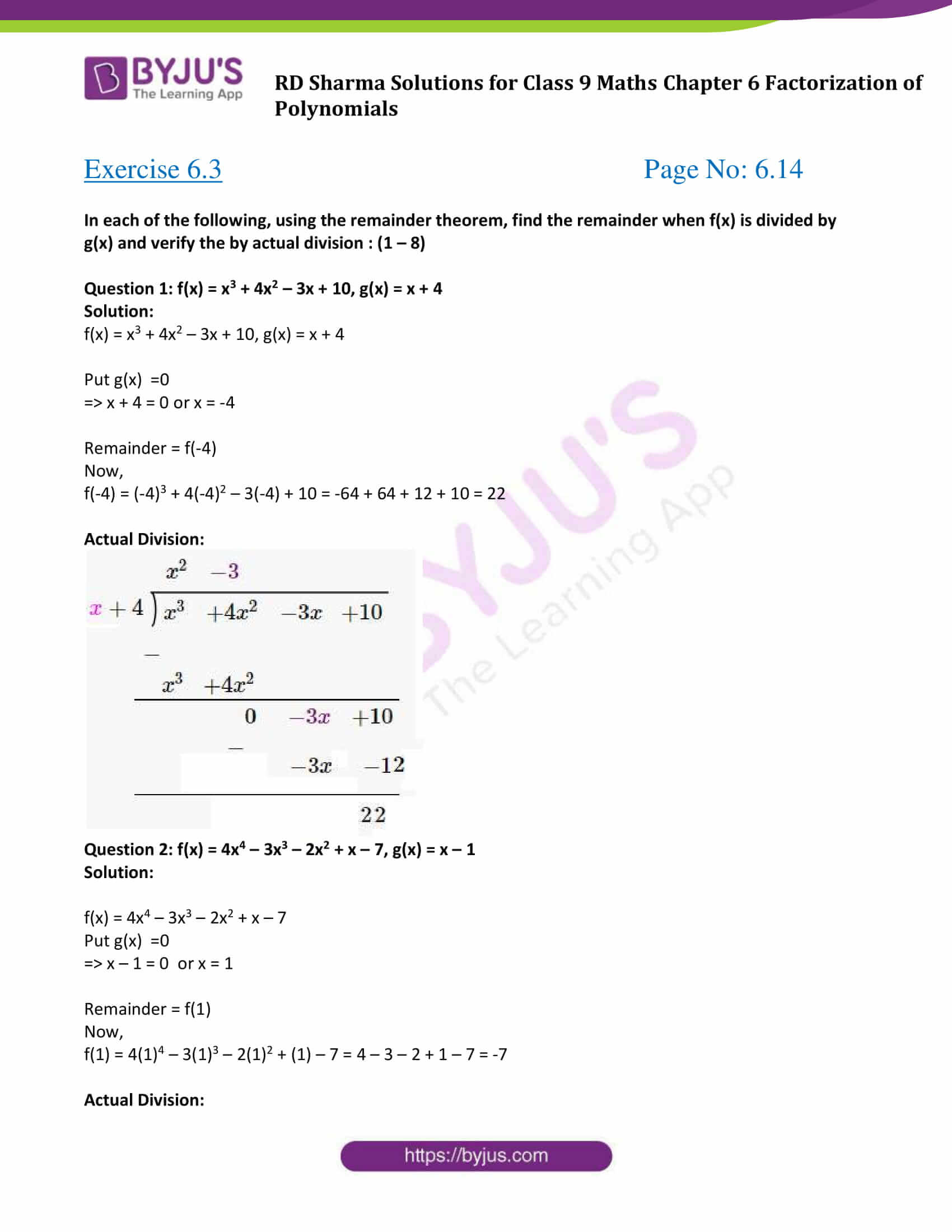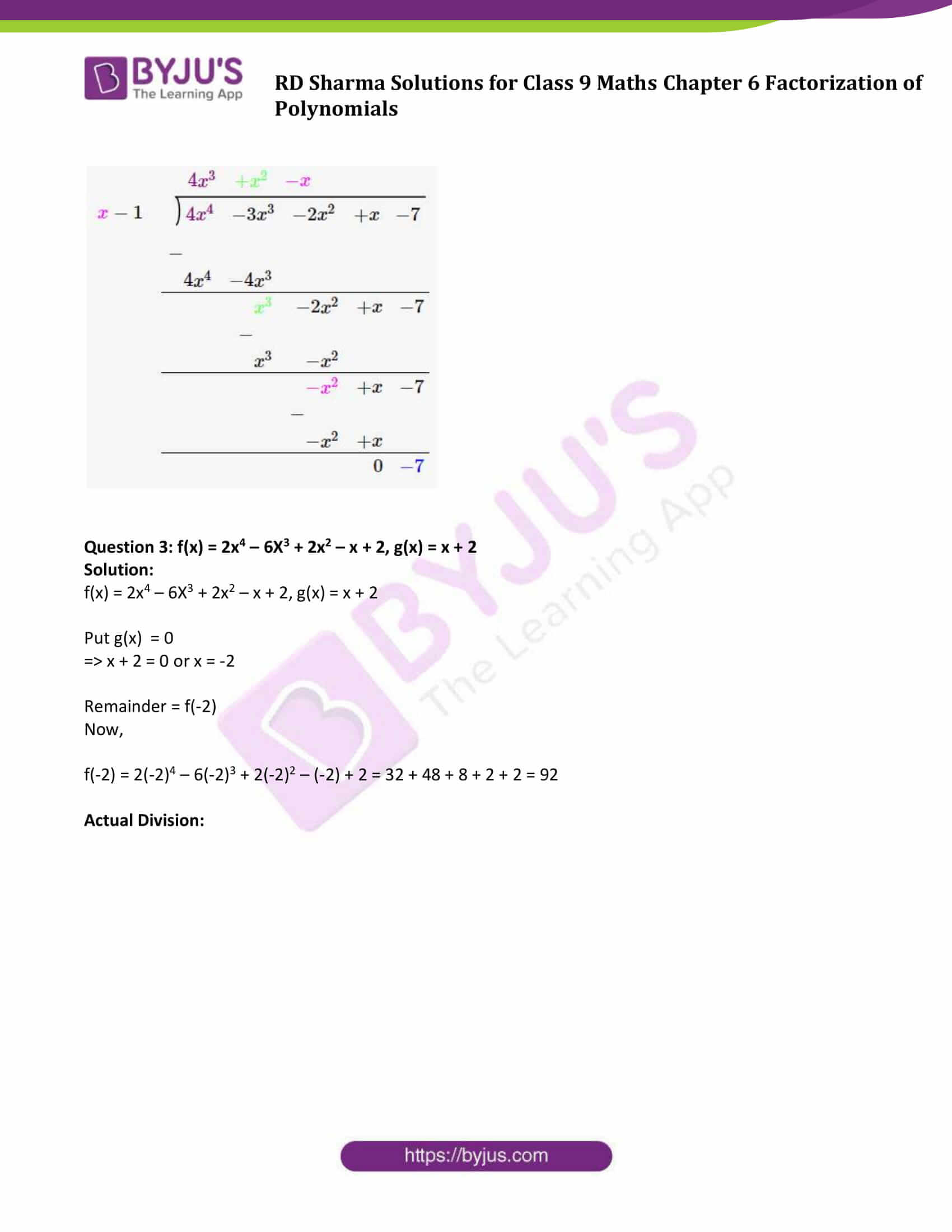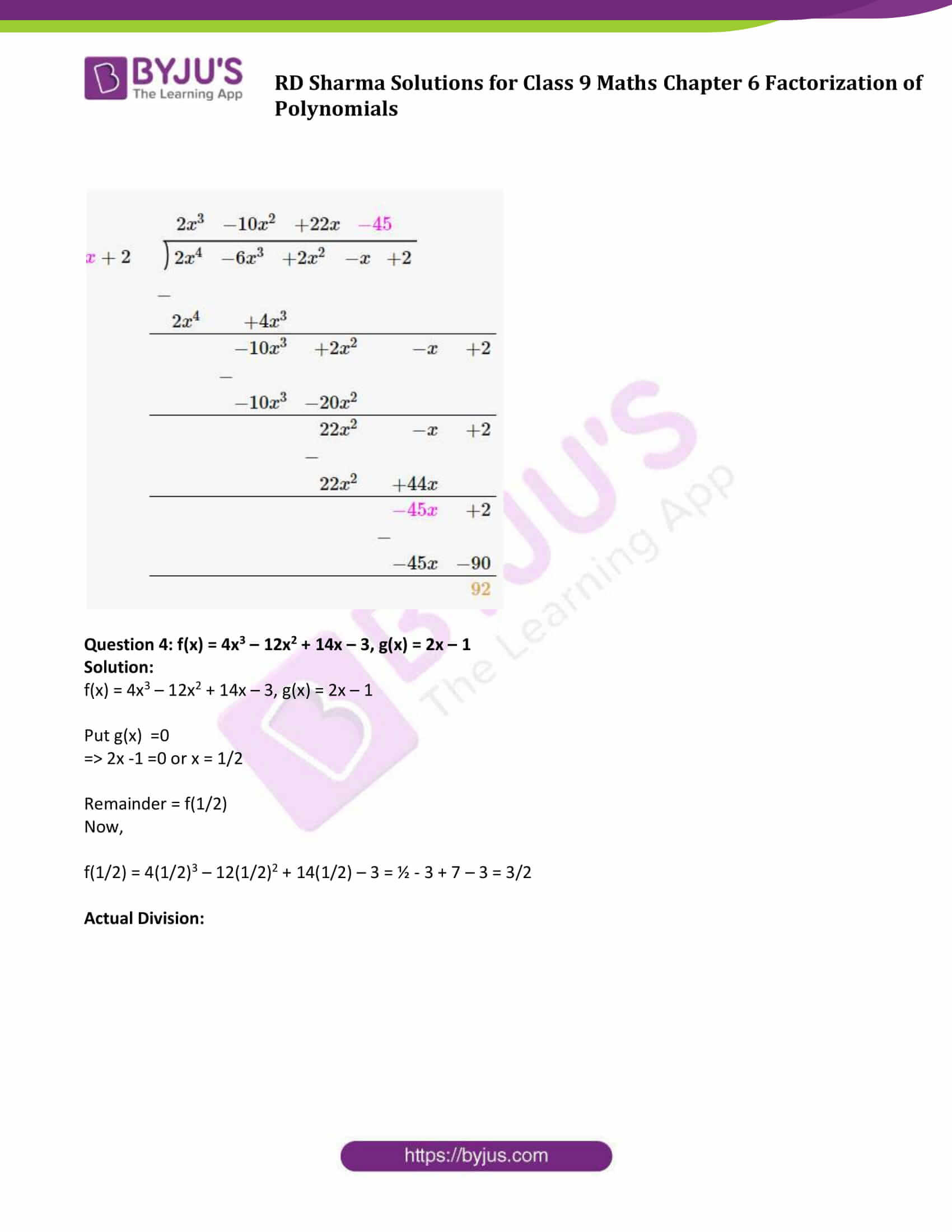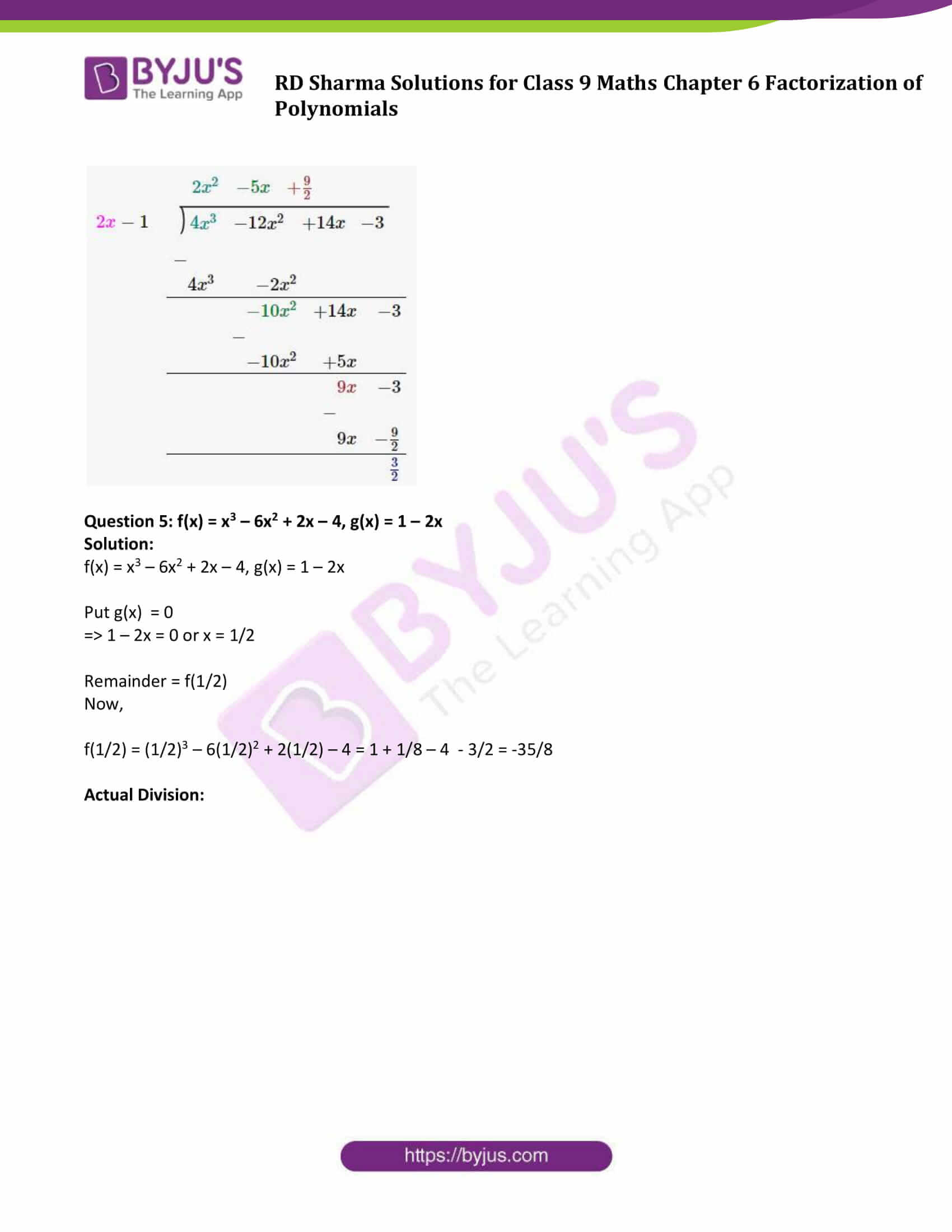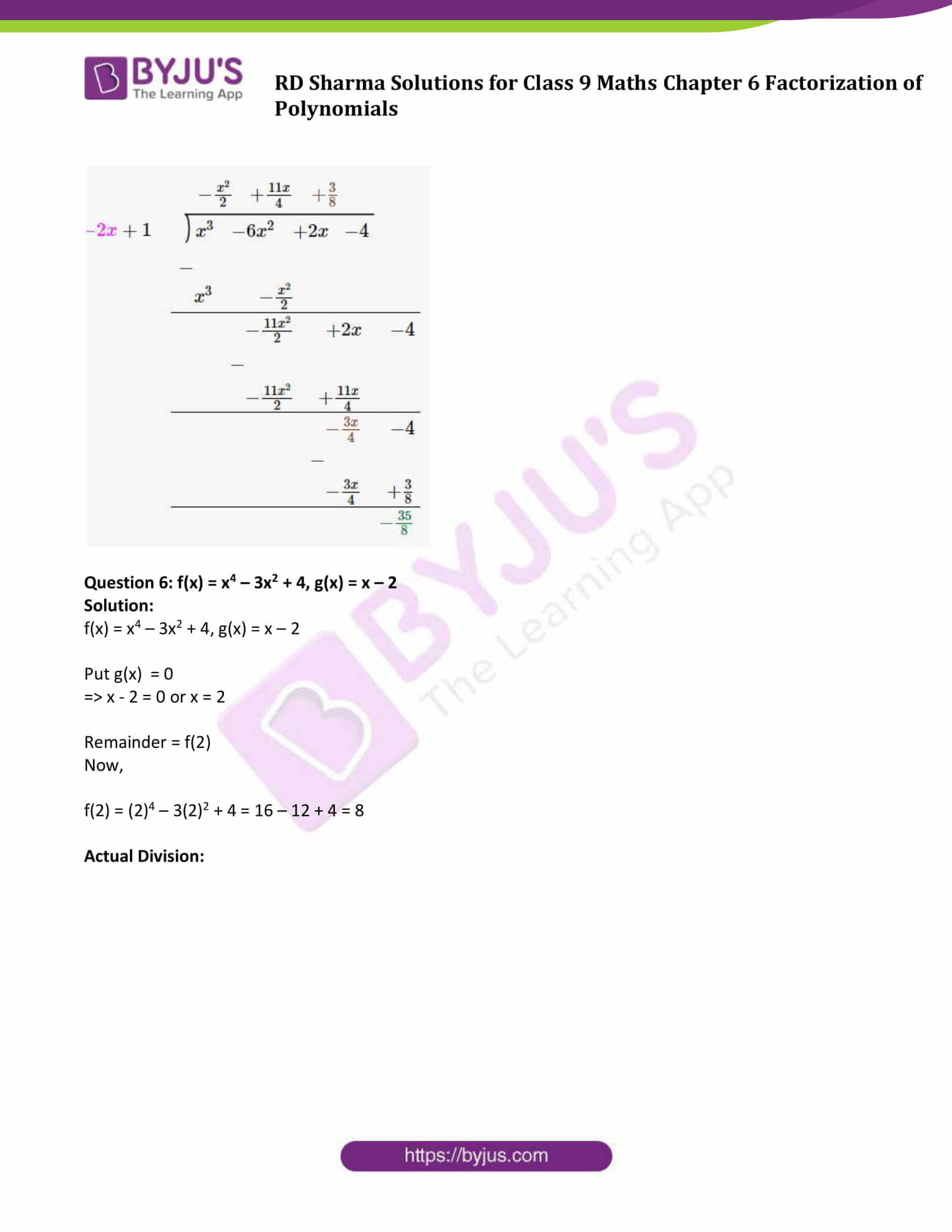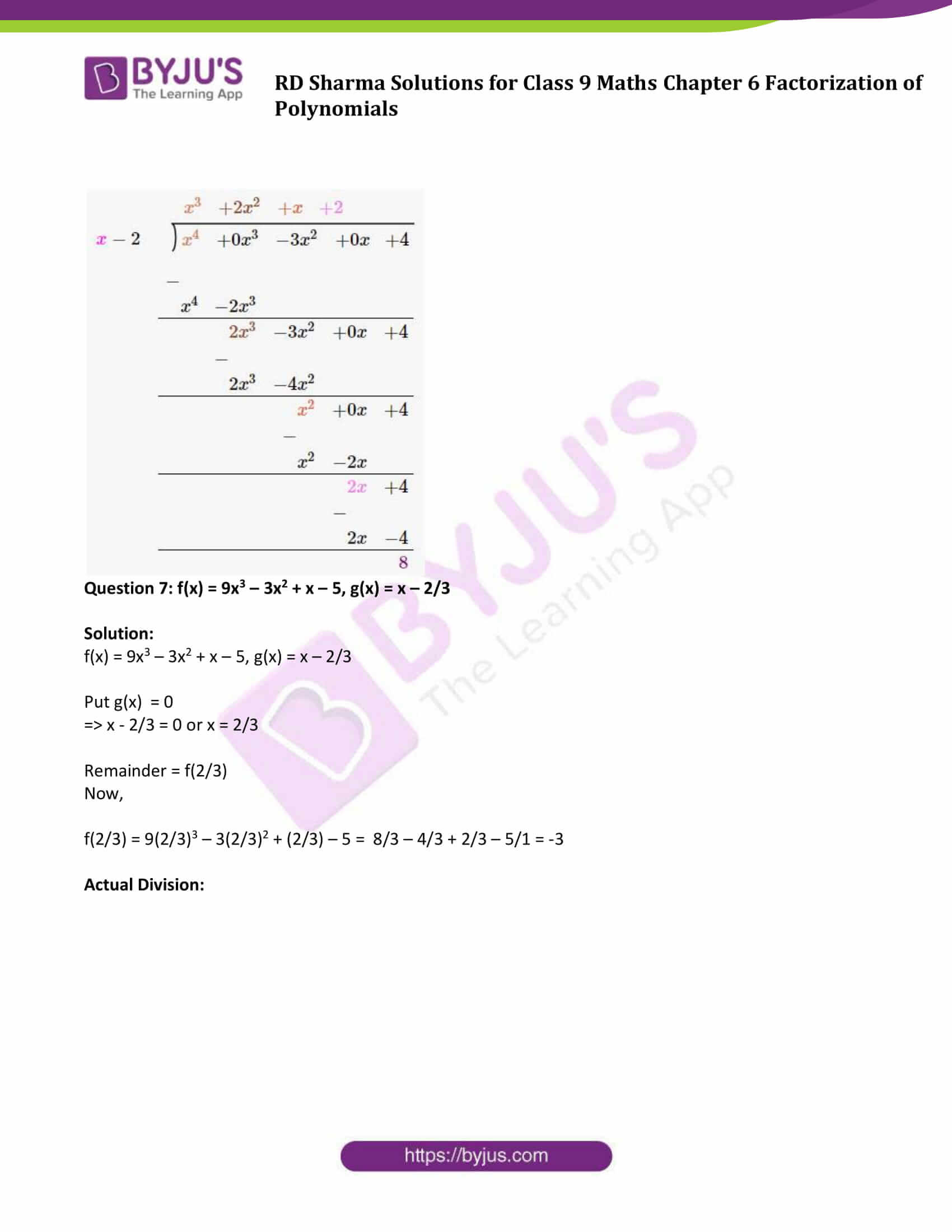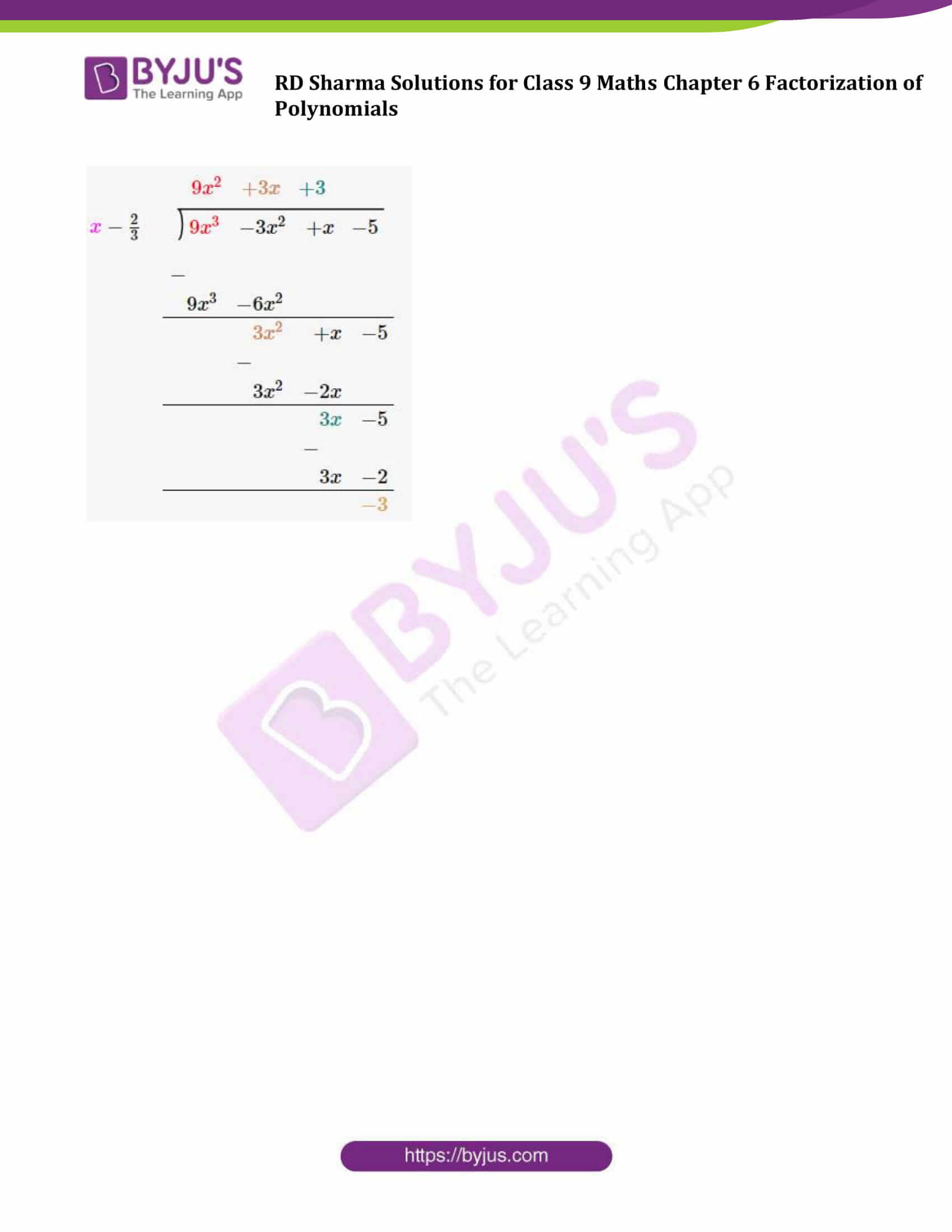### Access Answers to Maths RD Sharma Solutions for Class 9 Chapter 6 Factorization of Polynomials Exercise 6.3 Page number 6.14

#### Exercise 6.3 Page No: 6.14

In each of the following, using the remainder theorem, find the remainder when f(x) is divided by g(x) and verify the by actual division : (1 – 8)

Question 1: f(x) = x3 + 4x2 – 3x + 10, g(x) = x + 4

Solution:

f(x) = x3 + 4x2 – 3x + 10, g(x) = x + 4

Put g(x) =0

=> x + 4 = 0 or x = -4

Remainder = f(-4)

Now,

f(-4) = (-4)3 + 4(-4)2 – 3(-4) + 10 = -64 + 64 + 12 + 10 = 22

Actual Division: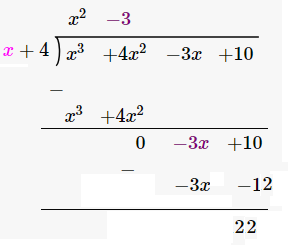Question 2: f(x) = 4x4 – 3x3 – 2x2 + x – 7, g(x) = x – 1

Solution:

f(x) = 4x4 – 3x3 – 2x2 + x – 7

Put g(x) =0

=> x – 1 = 0 or x = 1

Remainder = f(1)

Now,

f(1) = 4(1)4 – 3(1)3 – 2(1)2 + (1) – 7 = 4 – 3 – 2 + 1 – 7 = -7

Actual Division: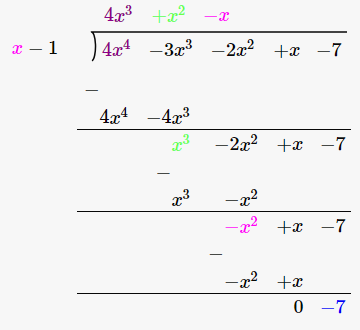Question 3: f(x) = 2x4 – 6X3 + 2x2 – x + 2, g(x) = x + 2

Solution:

f(x) = 2x4 – 6X3 + 2x2 – x + 2, g(x) = x + 2

Put g(x) = 0

=> x + 2 = 0 or x = -2

Remainder = f(-2)

Now,

f(-2) = 2(-2)4 – 6(-2)3 + 2(-2)2 – (-2) + 2 = 32 + 48 + 8 + 2 + 2 = 92

Actual Division: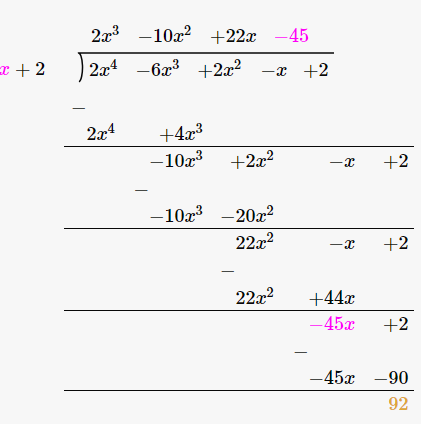Question 4: f(x) = 4x3 – 12x2 + 14x – 3, g(x) = 2x – 1

Solution:

f(x) = 4x3 – 12x2 + 14x – 3, g(x) = 2x – 1

Put g(x) =0

=> 2x -1 =0 or x = 1/2

Remainder = f(1/2)

Now,

f(1/2) = 4(1/2)3 – 12(1/2)2 + 14(1/2) – 3 = ½ – 3 + 7 – 3 = 3/2

Actual Division: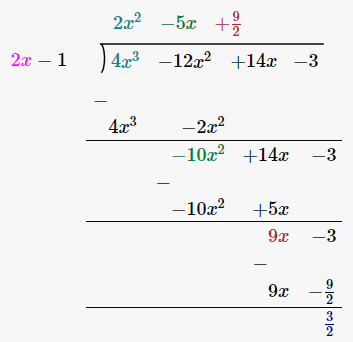Question 5: f(x) = x3 – 6x2 + 2x – 4, g(x) = 1 – 2x

Solution:

f(x) = x3 – 6x2 + 2x – 4, g(x) = 1 – 2x

Put g(x) = 0

=> 1 – 2x = 0 or x = 1/2

Remainder = f(1/2)

Now,

f(1/2) = (1/2)3 – 6(1/2)2 + 2(1/2) – 4 = 1 + 1/8 – 4 – 3/2 = -35/8

Actual Division:Question 6: f(x) = x4 – 3x2 + 4, g(x) = x – 2

Solution:

f(x) = x4 – 3x2 + 4, g(x) = x – 2

Put g(x) = 0

=> x – 2 = 0 or x = 2

Remainder = f(2)

Now,

f(2) = (2)4 – 3(2)2 + 4 = 16 – 12 + 4 = 8

Actual Division: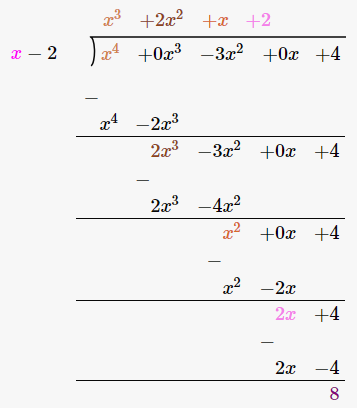Question 7: f(x) = 9x3 – 3x2 + x – 5, g(x) = x – 2/3

Solution:

f(x) = 9x3 – 3x2 + x – 5, g(x) = x – 2/3

Put g(x) = 0

=> x – 2/3 = 0 or x = 2/3

Remainder = f(2/3)

Now,

f(2/3) = 9(2/3)3 – 3(2/3)2 + (2/3) – 5 = 8/3 – 4/3 + 2/3 – 5/1 = -3

Actual Division: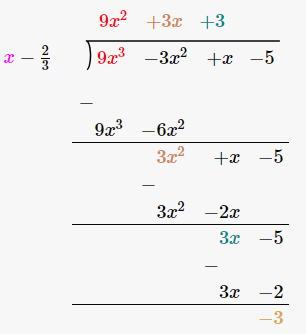## RD Sharma Solutions for Class 9 Maths Chapter 6 Factorization of Polynomials Exercise 6.3

RD Sharma Solutons Class 9 Maths Chapter 6 Factorization of Polynomials Exercise 6.3 is based on Remainder Theorem. RD Sharma solutions for class 9 are prepared by subject experts to help students in their endeavour to have a better understanding and score well in examinations.